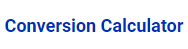#### Online Conversion Calculator :Conversion Calculator

##### Conversion Calculator :​
• You can easily use this online kVA to VA calculator for converting kVA to VA.
• Enter Kilowatt-amps.
• Click “Calculate” to get the result and then if you want to clear values Click “Reset” in addition to clear the values.
• Besides, the formula this calculator works.
• Finally, follow these steps to get the results.
• Then you will get as a result in Volt-amps (VA).
##### How it Works :
• If you choose the Length,
1. Inch.
2. Feet.
3. Meter.
4. Centimeter.
5. Kilometer.
6. Millimeter.
7. Yard.
8. Mile.
• If you choose the Temperature,
1. Fahrenheit.
2. Celsius.
3. Kelvin.
• If you choose the Weight,
1. Ounce.
2. Pounds.
3. Ton.
4. Metric Ton.
5. Milligram.
6. Gram.
7. Kilogram
• If you choose the Area,
1. Acre.
2. Hectare.
3. Square Meter.
4. Square Kilometer.
5. Square Centimeter.
6. Square Millimeter.
7. Square Inch.
8. Square Feet.
• These are the conversion calculator which are will be shown when you choose any conversion.
• If you choose any conversion calculator. For example,
• You choose Inch and enter the number then you will get other calculators result.
• These are the properties followed for balance conversion calculators.
• Each and every conversion have different types of calculators to convert the answer.
• These are the steps to be followed to get the result of conversion calculators.

##### Other Calculators :
Get it or Get it done!

Get a free Revit family by requesting it. For the first 100 persons* the next day.

*Conditions apply​

Hi Myself Raj,
Do you need any BIM Modelling Services